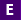#Not a Florida public school educator?

Access this resource
on CPALMS.com

### General Information

Subject(s): Mathematics, Science
Intended Audience: EducatorsSuggested Technology: Computers for Students, Internet Connection
Instructional Time: 60 Minute(s)
Keywords: rounding, Scratch, powers of 10, decimals, coding, computer science, programming, order of operations, divide by 10, multiply by 10
Instructional Component Type(s):

## Aligned Standards

This vetted resource aligns to concepts or skills in these benchmarks.

## 1 Lesson Plan

Rounding Decimal Numbers - Lesson #2

This is lesson 2 of 3 in the Rounding Decimal Numbers unit. This lesson introduces Traditional Rounding method to determine an estimated value rounded to a specific place value. This lesson bridges the concepts of fractions to decimals and powers of ten as well expanded notation to rounding methods.

This lesson uses flowchart model to describe the method in which the rounding is performed. Rounded numbers are verified using a Scratch activity in this lesson.

## Related Resources

Other vetted resources related to this resource.

## Lesson Plan

Rounding Decimal Numbers - Lesson #2:

This is lesson 2 of 3 in the Rounding Decimal Numbers unit. This lesson introduces Traditional Rounding method to determine an estimated value rounded to a specific place value. This lesson bridges the concepts of fractions to decimals and powers of ten as well expanded notation to rounding methods.

This lesson uses flowchart model to describe the method in which the rounding is performed. Rounded numbers are verified using a Scratch activity in this lesson.

Type: Lesson Plan